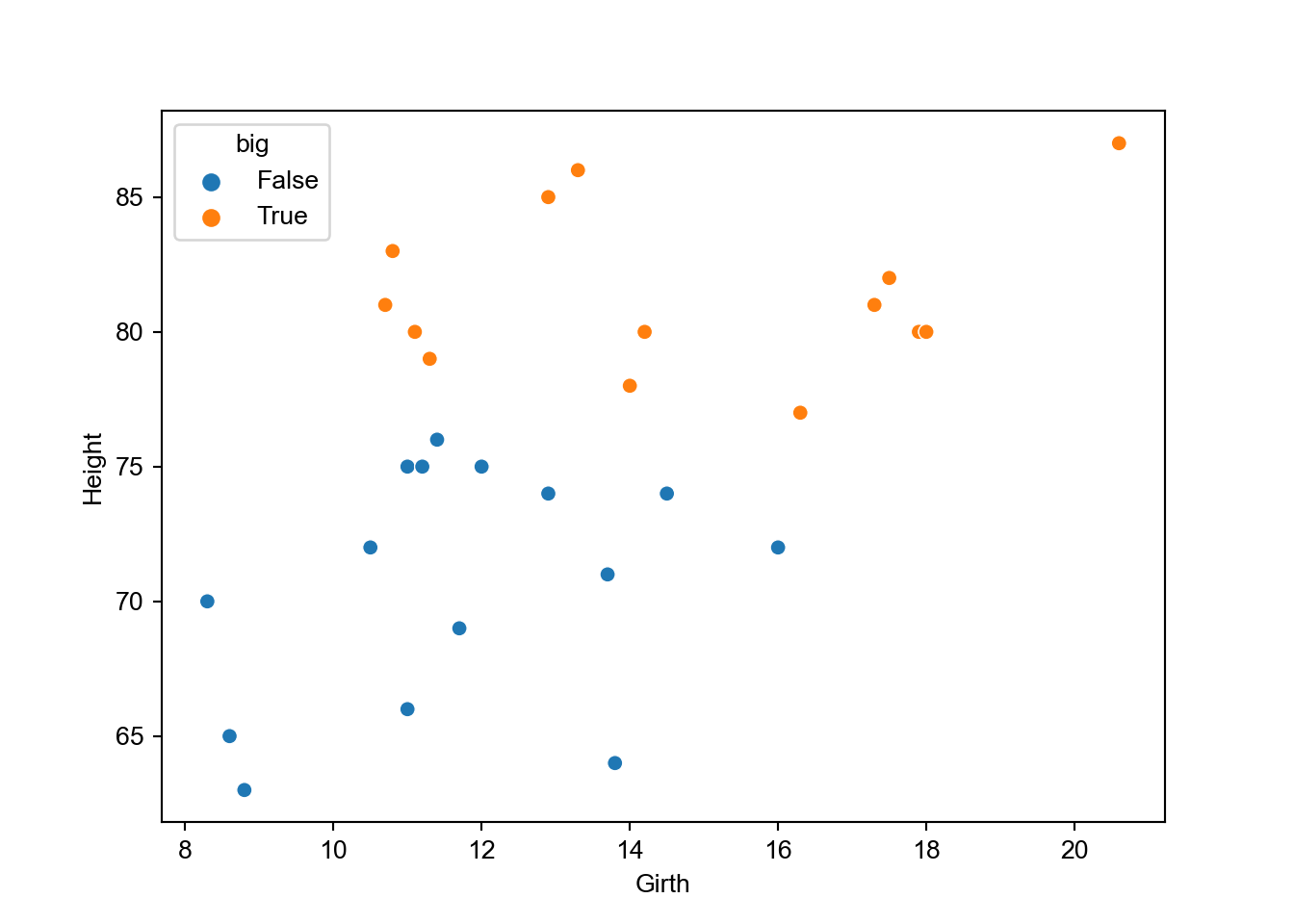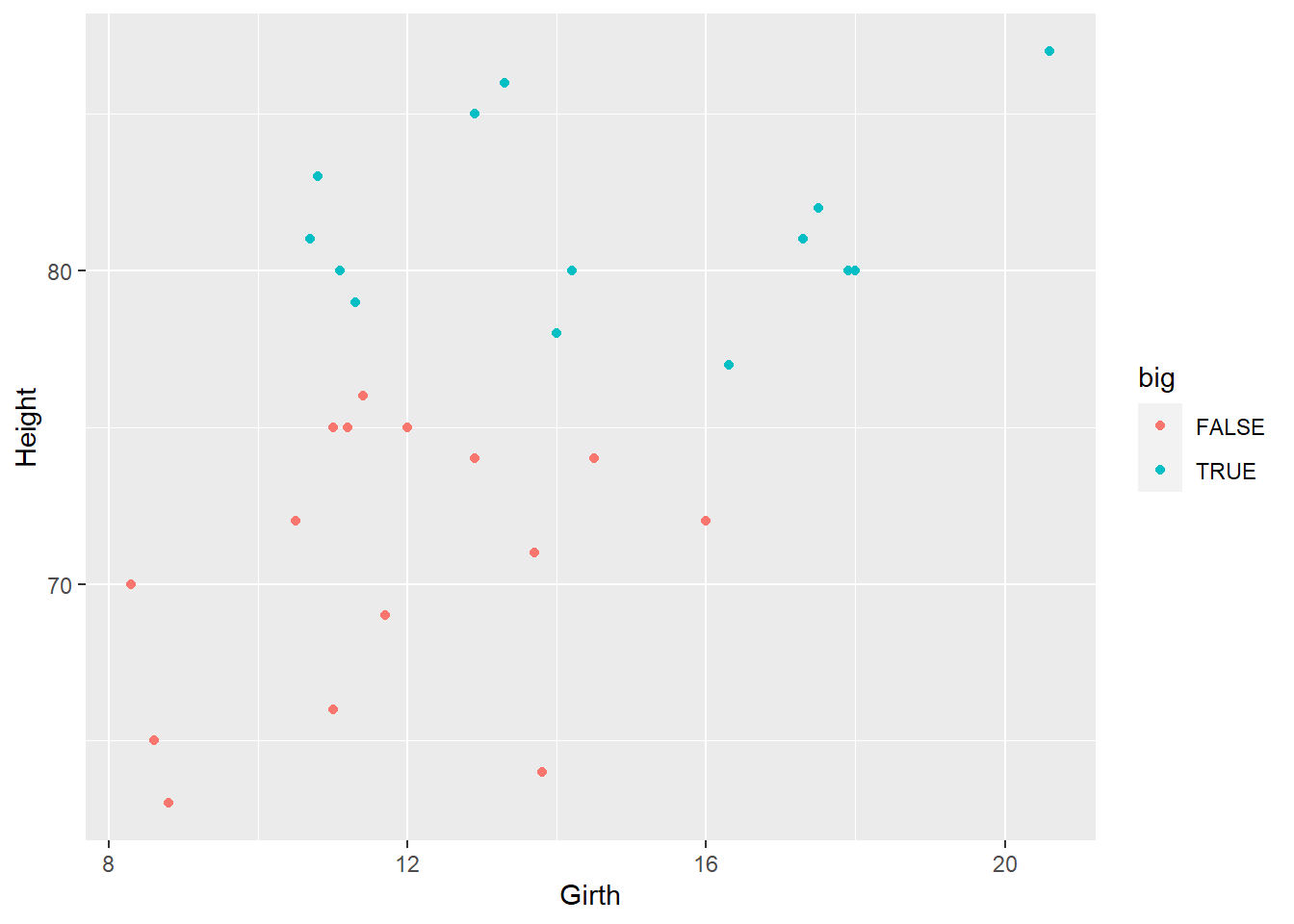# Python in R Markdown

An R package for writting `Python` code in R Markdown
How-to
R
Python
Rmarkdown
Author

Mark Edney

Published

March 3, 2022Photo by David Clode on Unsplash

The main advantage of using the R Markdown format is the utility of running R code within the text. This is clearly more advantageous than just writing code in a Markdown file. R Markdown is however limited to R code, unable to run Python scripts. The R library `reticulate` looks to add this capability.

## Initial Setup

The initial setup requires the installation of the `reticulate` library, after installation you shouldn’t need to call it, but I do in the preceding code. I have loaded the `trees` dataset as a test dataset and the tidyverse library just to explore the data a bit.

``````library(reticulate)
library(tidyverse)
data(trees)
glimpse(trees)``````
``````Rows: 31
Columns: 3
\$ Girth  <dbl> 8.3, 8.6, 8.8, 10.5, 10.7, 10.8, 11.0, 11.0, 11.1, 11.2, 11.3, …
\$ Height <dbl> 70, 65, 63, 72, 81, 83, 66, 75, 80, 75, 79, 76, 76, 69, 75, 74,…
\$ Volume <dbl> 10.3, 10.3, 10.2, 16.4, 18.8, 19.7, 15.6, 18.2, 22.6, 19.9, 24.…``````

Now, R Studio will use your local version of Python when you write any code in a code chuck labelled with the “{Python}” header. If you don’t have any local version, R Studio will ask if you would like to install Miniconda. From here, you will need to start downloading the required Python modules.

Modules can be downloaded with the `pip` python package installer from the terminal or command line. The easiest method in R Studio is within the terminal window next to the console window. The command used is `pip install "module name"`. Some modules can be tricky and won’t work if not installed after other modules.

``````import numpy as np
import pandas as pd
import matplotlib.pyplot as plt
import seaborn as sns``````

## Multiple Environments

After the setup, you should see some additional options in the environment in R Studio. You should see that you have the option to switch between the R and Python environments.

Data is transitioned from the R environment to the Python environment with the `r` variable. This method should pretty similar to the Shiny Apt’s use of `input\output`. It is not only data that can move between environments, but functions too.

The following code takes data from the R environment and creates a plot in `Seaborn`. The mean values of the columns are calculated in `python` to be imported into the R environment. A simple linear model is created with the `SKlearn` module.

``````data = r.trees
means = np.mean(data, axis = 0)
data["big"] = data.Height > means.Height
sns.scatterplot(data = data, x= "Girth", y= "Height", hue = "big")
sns.set_theme(color_codes=True)
plt.show()````````````from sklearn.linear_model import LinearRegression
mdl = LinearRegression()
mdl.fit(data[["Girth"]], data[["Height"]])``````
``LinearRegression()``
``print(mdl.coef_)``
``[[1.05436881]]``

Data is transitioned from `Python` to, `R` similarly with the variable `py`. Information on models can be passed but not the models themselves. This is important if you are more comfortable creating models in `Python`.

``print(py\$means)``
``````   Girth   Height   Volume
13.24839 76.00000 30.17097 ``````
``print(py\$mdl\$intercept_)``
`` 62.03131``
``````py\$data %>%
ggplot(aes(x = Girth, y = Height, colour = big)) +
geom_point()``````